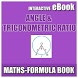# Maths Angle & Trigonometric Ratio Formula BookFree App

Rated 0.00/5 (0) —  Free Android application by Learn with Quiz

Advertisements

## About Maths Angle & Trigonometric Ratio Formula Book

Angle & Trigonometric Ratio Interactive e-Book for IIT-JEE & other Competitive Exams.

Board: CBSE, GSEB, ICSE, ISC, International Board, Cambridge
Class: 11th - 12th Students, Engineering Students and Medical Students.

Maths (AIEEE, IIT-JEE Main) Angle & Trigonometric Ratio Interactive e-Book Education application

### How to Download / Install

Download and install Maths Angle & Trigonometric Ratio Formula Book version 1.0 on your Android device!
Downloaded 10+ times, content rating: Not rated
Android package: com.wMathsAngleandTrigonometricRatioFormulaBook_5147311, download Maths Angle & Trigonometric Ratio Formula Book.apk

### All Application Badges

Free
downl.
Android
2.3+
n/a
Not
rated
Android app

Oh snap! No comments are available for Maths Angle & Trigonometric Ratio Formula Book at the moment. Be the first to leave one!

RATING
0.05
0 users

5

4

3

2

1